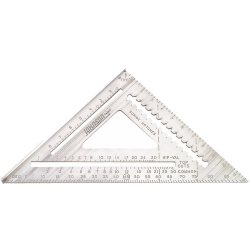# Carpentry Tools: Rafter Angle Squares

## Rafter Angle Square How To's

View all construction squares from Johnson Level.

## Rafter Angle Squares

Speed the job of rafter or angle layout by using our Rafter Angle Square, also known as a square. With one number on our square you can frame a roof or a solar panel support, or layout stairs, cabinets, trim work, or many other construction projects.

Johnson's Rafter Angle Square has a handy 6" rule for quick measurements and scribing. The easy-to-read angle scale allows you to find any angle from 0 degrees to 180 degrees for many of your special designs such as carvings, picture framing, furniture, room dividers, shadow boxes, "cut-outs", routings and more. The rafter scales are laid out mathematically to take the time-consuming calculations out of your layout work. This gives you the accuracy you need with the convenience of using one number to work with. The Rafter Angle Square also makes an excellent right angle guide for an electric saw to run against.

Both the professional tradesman and the handyman will find the Rafter Angle Square convenient to use. All of the necessary instructions for basic roof construction are included in our accompanying PDF, along with other uses of the square such as stair layout and the framing of a solar panel support. An abundance of descriptive drawings and tables have been added, as well as an index for rapid access to each section.## How to Read a Rafter Angle Square

1. Find the lip edge. This edge keeps the rafter angle square from lying flat. Use it as a straight edge.
2. Locate the hypotenuse, running at a 45 degree angle from the legs.
3. Follow the lip and adjacent leg to where they form a 90 degree angle. This is called the pivot.
4. Locate the common label along the long inner cutout. This measures pitch (generally used for roof pitch).
5. Find the degrees along the hypotenuse. Use this measurement as an alternative to common.

## How to Use a Rafter Angle Square

1. Lay the square on the surface of the material so the lip extends down the long side of the material.
2. Rotate the lip away from the edge of the material around the pivot. The corner where the hypotenuse and lip meet should move, not the pivot.
3. Line up the desired angle, as indicated on the hypotenuse, on the same edge as the pivot.
4. If you are measuring by pitch as opposed to angles, line up the desired pitch, as indicated by the common edge, on the same edge as the pivot.
5. Mark along the outside edge of the rafter angle square for your angled cut.

## How to Use a Rafter Angle Square for Roof Pitch

1. Find a common rafter angled with the pitch of the roof.
2. Use a spirit level to mark a straight vertical line across the rafter. This is called the plumb line.
3. Align the lip of the rafter angle square along the plumb line.
4. Line up the pivot point on the bottom edge of the rafter.
5. Follow the bottom edge of the rafter to where it meets the common pitch reading.
6. The corresponding value measures the pitch of the roof.

## How to Use a Rafter Angle Square for Rafters

1. Put the square against the surface of the material you want to use. Mark the location of the diagonal edge. That will create a 45° line.Using the pivot point (the notch on the non-lip leg of the square), adjust the square until the degree gradation you need lines up with the edge. Draw a pencil line along the square on the surface.
2. Press the square against the edge. Place a pencil tip into the notch to measure the number of inches you need. Keep the pencil in the notch and sli
3. de the square along the material. You’ll get a straight line parallel to the edge.
4. Position the square flat on the board you want to cut. Slide the saw shoe against the square. The rafter angle square is thicker so the saw cut will be precise.

## How to Cut Rafters with a Rafter Angle Square

1. Determine the building’s width and length and the
rafter span in order to evaluate how many rafters are needed. Then determine the pitch. Divide the rafter’s span by two to find the rafter’s run. As an example, a building that’s 24 feet wide has a rafter run of 12. If your rise is 5, the pitch is 5/12. Spacing the rafters 24 inches apart, you’ll need 15 rafters for a 30-foot long building.
2. Mark the top (the plumb) first. Put the pivot point, where the square’s straight edge meets the lip side, at the rafter board’s top edge. Rotate the square until the correct pitch is in line with the board’s edge. Using the opposite side of the square, draw a line down the side of the board. This is your plumb line. After the rafter length is set and cut, make another cut 3/4 inch from plumb to allow for two-inch ridge board for where rafters meet.
3. Find the rafter length needed by consulting the rafter table inside of therafter angle square manual. Returning to our example, a 5/12 inch rafter has a length of 13 inches.
4. Find the birdsmouth joint.